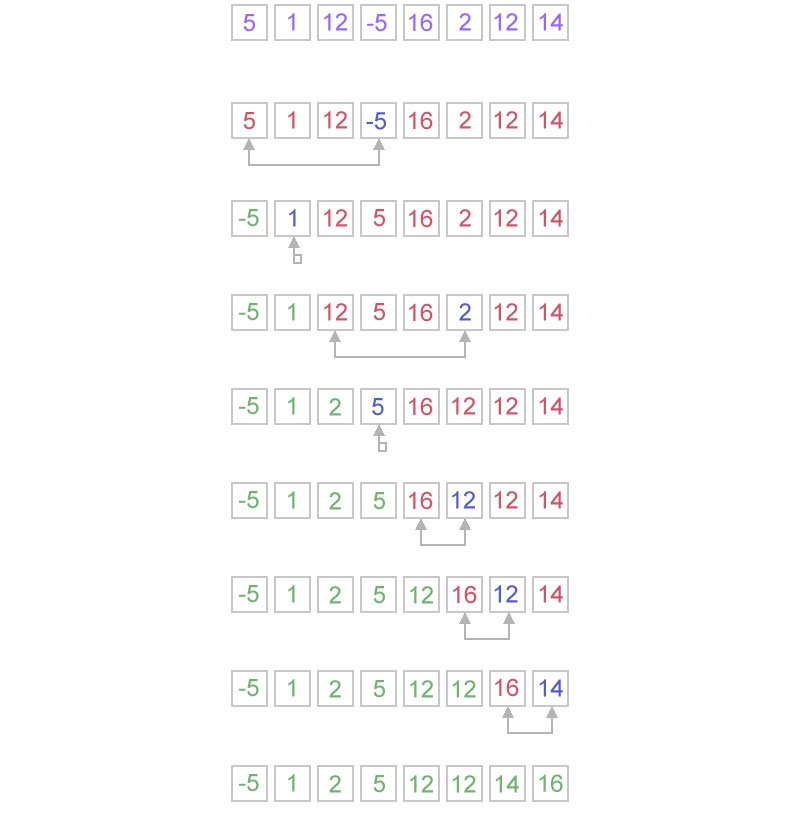## Selection Sort

Selection sort is a sorting algorithm that uses a combination of searching and sorting to complete the task. During each pass, the unsorted element with the largest (or smallest) value is moved to its proper position in the array. The number of times the sort passes through the array is one less than the number of items in the array. In the selection sort, the inner loop finds the next largest (or smallest) value and the outer loop places that value into its proper location.## In-place Sort

The array is sorted in-place, so there is no need for extra memory or a temporary array. One important point about the selection sort algorithm is that it is a brute-force sorting algorithm. If the array is sorted before the algorithm is run, it doesn’t matter. It will still go through all the comparisons, all the loops, etc. Nothing will ever get switched, but it will make all the comparisons never-the-less. So it doesn’t matter what the sorted state of the original array is, the sorting using selection sort will cost exactly the same.

## Selection Sort Complexity

Selection sort is noted for its simplicity, and also has performance advantages over more complicated algorithms in certain situations. Selection sort is a quadratic sorting algorithm since it has worst-case and average complexity both О(n2), where n is the number of items being sorted.. Selecting the largest (or smallest) element requires scanning all n elements (this takes n − 1 comparisons) and then swapping it into the last ( or smallest) position. Finding the next highest or lowest element requires scanning the remaining n − 1 elements and so on, for (n − 1) + (n − 2) + … + 2 + 1 = n(n − 1) / 2 ∈ Θ(n2) comparisons. Each of these scans requires one swap for n − 1 elements.

## Selection Sort Algorithm

Below is an example of Selection Sort written in Java..

``` package miftyisbored;

/**
*
* @author m_yusuf
*	This is a small class that implements Selection Sort
*/
public class MiftySelectionSorter{
/**
* This main method is used to illustrate how to use the Selection Sort Algorithm
**/
public static void main(String[ ] args)
{
final String BLANK_SPACE = "  "; // Just because I am lazy 🙂
int[ ] data = { 10000, 1002, 83, 12, 58, 72, 66, 65, 75648, 90, 23, 37, 41, 66, 0, 3100, 55555, 1, 67 };

// Print the array before sorting:
System.out.println("Welcome to Mifty's Selection Sorter");
System.out.println("Here is the original unsorted list:");
for (int i = 0; i < data.length; i++)
System.out.print(data[i] + BLANK_SPACE);
System.out.println( );

// Sort the numbers, and print the results
selectionSort(data);
System.out.println("All numbers are sorted now!");
System.out.println("Here is the ordered list:");
for (int i = 0; i < data.length; i++)
System.out.print(data[i] + BLANK_SPACE);
System.out.println( );
}

/**
* This function sorts an array of integers from smallest to largest using selection sort
* @param: data - the array to be sorted
*/
public static void selectionSort(int[ ] data){

int length = data.length;  // get the size of elements
int smallestIndex;  // Index of smallest value in data...data[i]
int tempValue; // Used during the swapping of two array values

for (int i = 0; i < length; i++){
// find the largest element and place it the end
smallestIndex = i;
for (int j = i+1; j < length; j++){
if (data[smallestIndex] > data[j]){
smallestIndex = j;
}
}

// swap data[i] with data[smallestIndex]:
tempValue = data[i];
data[i] = data[smallestIndex];
data[smallestIndex] = tempValue;
}
}
}

```

The following is the output of the application

```Welcome to Mifty's Selection Sorter
Here is the original unsorted list:
10000  1002  83  12  58  72  66  65  75648  90  23  37  41  66  0  3100  55555  1  67
All numbers are sorted now!
Here is the ordered list:
0  1  12  23  37  41  58  65  66  66  67  72  83  90  1002  3100  10000  55555  75648
```

Mifty Yusuf is a Montreal-based software developer who enjoys playing with new web technologies as well as comic books and illustrations. He beleives that, no matter what the question is, the answer is always Batman!

## 1 Comment

1. […] generally performs better than quadratic sorting algorithms such as bubble sort, insertion sort and selection sort. But unlike these other sorts, which take list and modify it in place, merge sort requires […]

This site uses Akismet to reduce spam. Learn how your comment data is processed.

Go to Top